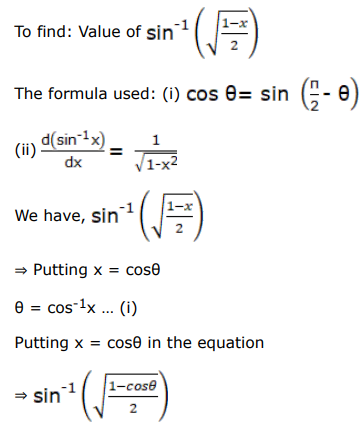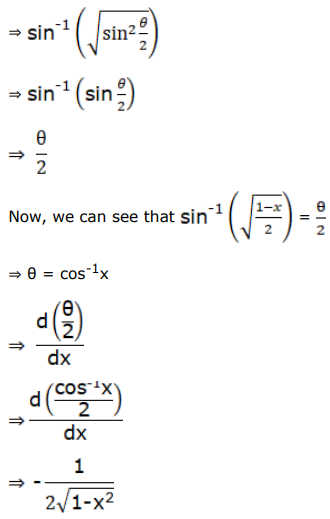# Differentiate each of the following w.r.tQuestion:

Differentiate each of the following w.r.t $x$ :

$\sin ^{-1}\left(\sqrt{\frac{1-x}{2}}\right)$

Solution:$A n s)=\frac{1}{2 \sqrt{1-x^{2}}}$